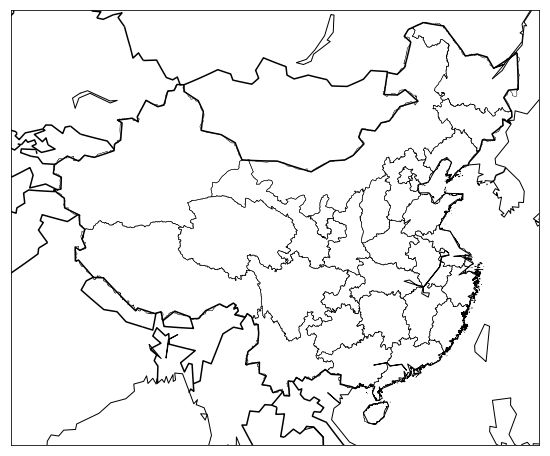# 用Python画一个中国地图## Pandas

``````    df = pd.read_csv('a.csv')
``````

## 安装 Basemap

``````    brew install geos
pip3 install https://github.com/matplotlib/basemap/archive/v1.1.0.tar.gz
``````

## 开始画图

``````import matplotlib.pyplot as plt
from mpl_toolkits.basemap import Basemap

plt.figure(figsize=(16,8))
m = Basemap()
m.drawcoastlines()

plt.show()
````````````    m.drawcountries(linewidth=1.5)
```````Java`可能吗？用`PHP`可能吗？

``````    m = Basemap(llcrnrlon=73, llcrnrlat=18, urcrnrlon=135, urcrnrlat=53)
````````````    m = Basemap(llcrnrlon=77, llcrnrlat=14, urcrnrlon=140, urcrnrlat=51, projection='lcc', lat_1=33, lat_2=45, lon_0=100)
````````````m.readshapefile('CHN_adm_shp/CHN_adm1', 'states', drawbounds=True)
``````